## USAMO

Go back to the Math Jam Archive

We will discuss the problems from the second day of the 2004 USAMO.

#### Facilitator: Mathew Crawford

Welcome to the USAMO Day 2 Math Jam.

MCrawford (19:00:24)
We will begin with problem 4.

MCrawford (19:00:29)
4. Alice and Bob play a game on a 6 by 6 grid. On his or her turn, a player chooses a rational number not yet appearing in the grid and writes it in an empty square of the grid. Alice goes first and then the players alternate. When all squares have numbers written in them, in each row, the square with the greatest number in that row is colored black. Alice wins if she can then draw a line from the top of the grid to the bottom of the grid that stays in black squares, and Bob wins if she can't. (If two squares share a vertex, Alice can draw a line from one to the other that stays in those two squares.) Find, with proof, a winning strategy for one of the players.

MCrawford (19:00:50)
What facts do we have to play with?

Nukular (19:01:13)
Games often have a solution in which one person proceeds based on what the other person does.

MCrawford (19:01:45)
What other facts do we have?

MCrawford (19:02:51)
Bob always moves after Alice. What does this mean?

RK7 (19:02:59)
he can always counter her move

RK7 (19:03:10)
because there is an even number of rows and columns

jelyman (19:03:10)
bob responds to her moves and therefore decides which one of 2 places the highest number in a row can be

Nukular (19:03:15)
There are an even number of squares, and Bob can move based on what alice does.

MCrawford (19:03:33)
Since Bob can always follow Alice's moves, Bob has a certain amount of control over which number is the greatest in any given line. He can if he chooses always have the last word. How can we use this fact?

RK7 (19:04:19)
try to develop a strategy where the black squares are always separated by at least two horizontal squares

Nukular (19:04:23)
Make bob ""copy"" the moves of alice, only shifted down (or up) by one row, and over (left or right) by 2 or 3 columns. That way the black squares are ""separated"" and no line can connect them.

jelyman (19:04:45)
Make it so that the last slot (bob's) and the next highest are never accessible to the same adjacent squares on the row above/below

MCrawford (19:05:12)
For each row, Bob can make a choice at least 3 times. Suppose Bob always acts in the row Alice just acted in, can Bob use his 3 choices to derail Alice?

jelyman (19:05:36)
yes

MCrawford (19:05:47)
Each time Bob makes a choice, he can eliminate a square in the grid from becoming black. He does this either by placing a number in that cell smaller than the number Alice just placed in another cell, or by placing a higher number in a cell, eliminating the cell that Alice just played in from becoming black.

MCrawford (19:05:56)
Bob could decide even before the game starts to eliminate any 3 squares from becoming black. If Alice plays in one of those squares, he simply chooses one of the other 3 for placement of a higher number than Alice played. Otherwise, if Alice played in one of the 3 he didn't choose, he plays a smaller number than Alice played in one of the 3 he chose beforehand. Can Bob use this ability to guarantee a win?

Nukular (19:06:50)
Yes; choose 3 squares to prevent from being black, and make sure they dont line up from row to row.

MCrawford (19:07:03)
Bob can quite easily eliminate enough squares before the game starts to keep Alice from winning. All he must do is choose 3 squares per row in such a way as to cut off any path Alice could take down the grid:

MCrawford (19:07:08)MCrawford (19:07:15)
As we can see, the need only have three rows in order to allow a winning strategy for Bob (the top three in this case).

MCrawford (19:07:34)
5. Let a, b, and c be positive real numbers. Prove that

MCrawford (19:07:38)MCrawford (19:07:47)
Before we get too far into this problem I would like to mention that I plan to discuss two solutions here because this problem is most easily solved by a famous inequality that will be obscure to most students who are new to the USAMO. The first solution we will discuss does not make use of this famous inequality.

MCrawford (19:08:01)
First, what are the interesting features of this inequality?

RK7 (19:08:10)
cyclic symmetry

MCrawford (19:08:29)
What else?

RK7 (19:08:58)
something looking like the arithmetic mean on the right hand side

MCrawford (19:09:15)
Anything else (think simple)?

MCrawford (19:09:54)
The left hand side of this inequality is broken into three pieces and the right hand side is the product of three terms that are all the same. When pieces of an inequality are broken up into an equal number of products it is rare that we will not be comparing these products in some way -- either directly or through some inequality.

MCrawford (19:10:06)
Unfortunately the pieces on the left don't contain any structures that lead us to an obvious solution (that is, not obvious unless you're a highly experienced solver of inequalities).

We might also notice that there is equality at a = b = c = 1.

MCrawford (19:10:18)
Where might we begin to attack this inequality?

RK7 (19:10:45)
a^5 - a^2 + 3 ? a+b+c

Nukular (19:10:52)
Make it "nicer" looking. The fifth powers dont look nice.

MCrawford (19:11:04)
The right hand side is the cube of the sum of the variables. This is important because it makes us think of the power mean inequality chain

MCrawford (19:11:08)MCrawford (19:11:15)
The sum divided by 3 would give us the arithmetic mean. Unfortunately for us this mean must be less than or equal to the other side of the inequality, so the only part of the inequality chain we could use would be the RMS-AM inequality and the left hand side of our inequality looks nothing at all like the root mean square of three variables.

MCrawford (19:11:35)
We might next compare factors of the right and left hand sides directly:

MCrawford (19:11:39)MCrawford (19:11:56)
We can quickly abandon this path because the inequality that we would like to use is not even true. We can see this by taking small positive a and much larger values of b or c.

MCrawford (19:12:13)
Experienced solvers might at this point recognize the possible use of a third expression that we could sandwich in between the left and right hand sides. Perhaps an examination of one of the sides of the inequality will point us in the direction of such an expression. Which half of the inequality seems more likely to yield something interesting?

jelyman (19:12:41)
left cuz of the uniqueness

MCrawford (19:12:54)
The left hand side of this inequality is the unique part. If anything is going to provide us with a unique direction to work in, it's the unusual piece. What do we know that might help us work with the left hand side?

RK7 (19:13:34)
maybe add or subtract something so it factors?

MCrawford (19:14:00)
Do we have any clues as to how it might factor (in a convenient way)?

Nukular (19:14:12)
the fifth and second powers. work with those.

jelyman (19:14:14)
a^2 (a^3 -1)

jelyman (19:09:24)
c^2 * c^3 = c^5

MCrawford (19:14:33)
It is often extremely helpful to make seemingly useless observations about a problem, but the smallest piece of information can often point us in the right direction. We think that equality occurs when each of the variables is 1 and nowhere else. This makes us think to view the left hand side in terms of the number 1. If it factors, we can expect 1 to be a solution to at least one factor.

MCrawford (19:14:57)
The pieces of the left hand side don't seem to have any nice factorization, so we might think to add something that gives us a factorization that includes a root of 1.

MCrawford (19:15:12)RK7 (19:15:27)
so, if we make it 1 instead

RK7 (19:15:29)
we have

RK7 (19:15:57)
(a^2-1)*(a^3-1) +2 +a^3

MCrawford (19:16:08)
Of course, there are an infinite number of functions that we could add to this expression that would make 1 a factor, but it's likely that we'll use something relatively simple that we can most easily relate to the right hand side of the inequality. Seeing how we drew a^3 - 1 out of part of the expression,

MCrawford (19:16:27)
Drawing a^3 - 1 out of part of the expression gives us the clue to include a term involving a^3 in our expression. In fact, if we subtract a^3 from the expression we get

MCrawford (19:16:33)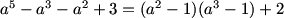Nukular (19:16:47)
(a^2-1)(a^3-1) >= 0.

MCrawford (19:17:05)
We now have two factors (a^2 - 1) and (a^3 - 1). These factors are both monotonically increasing functions for positive values of a. They both have one positive root where a = 1. This means that the two factors never have opposite signs. What does this mean?

Myself (19:17:27)
the left side is always positive

jelyman (19:17:31)
always pos

RK7 (19:17:33)
yes, it means their product is always positive

Nukular (19:17:26)
a^5 -a^2 + 1 >= a^3.

MCrawford (19:17:49)
We now have a nice inequality that might take us somewhere:

MCrawford (19:17:54)MCrawford (19:18:10)
How might we use this inequality?

RK7 (19:18:17)
multiplying out?

Nukular (19:18:17)
Plug that into the LHS.

MCrawford (19:18:29)
We might now try to sandwich pieces that look like the right hand side of this inequality in between the two sides of our original inequality:

MCrawford (19:18:37)MCrawford (19:18:46)
Can we prove the right half of this inequality chain?

RK7 (19:19:01)
oh, that looks like a really ugly inequality from the APMO

MCrawford (19:19:49)
Sometimes inequalities look ugly when they contain a lot of terms, but sometimes we can find ways to deal with all the terms and once we learn to approach inequalities this way, lots of terms don't necessarily look so ugly.

Nukular (19:19:56)
Here you might want to mulitply things out.

MCrawford (19:20:05)
We can expand

MCrawford (19:20:11)MCrawford (19:20:17)
How can we compare the two sides of this potential inequality?

Nukular (19:20:53)
AM-GM individual terms where we can.

MCrawford (19:21:21)
The left hand side has terms with 0, 3, 6, and 9 total powers of variables whereas everything on the right hand side has exactly 3 powers of variables. We can first subtract like terms from both sides:

MCrawford (19:21:29)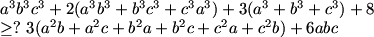MCrawford (19:21:38)
Now, the right hand side has terms like (a^2)b and abc. How can we compare these with terms on the left hand side?

RK7 (19:22:12)
ordering things?

MCrawford (19:22:29)
In what sense?

RK7 (19:22:33)
a>=b>=c

MCrawford (19:22:52)
That's often helpful, but in this case we don't need it.

Nukular (19:22:34)
(a^3+a^3+b^3) >= 3a^2b

MCrawford (19:23:36)
That might help us, but I'm going to advocate a different approach.

MCrawford (19:24:12)
The hardest terms to deal with are those on the LHS with total exponents of 9. How can we use those in AM-GM to compare them with terms with total exponents of 3?

Nukular (19:25:07)
combine w/ the constants

MCrawford (19:25:25)MCrawford (19:25:39)
How can we continue (dealing with hardest terms first)?

RK7 (19:25:58)
taking terms two at a time?

MCrawford (19:26:26)
We'll do some of that later (once we've organize our approach).

MCrawford (19:26:49)
How can we use terms like a^3b^3?

Nukular (19:27:21)
Combine them with terms like a^3

MCrawford (19:27:30)
How exactly?

MCrawford (19:28:16)
We need the 'exponential total' to add to 9 each time so that we can have a GM that fits the right hand side of the inequality.

RK7 (19:28:10)
oh, alright, so a^3+a^3b^3>= 3*a^2b

MCrawford (19:28:27)
Close.

MCrawford (19:28:35)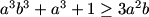Nukular (19:28:38)
a^3b^3 + a^3 + 1 >= 3a^2b

MCrawford (19:29:06)
What we are left with is the first suggestion that was offered:

MCrawford (19:29:13)MCrawford (19:29:21)
Ultimately we have 6 versions of the second inequality using a, b, and c in various combinations. If we hadd this to the first and third of these inequalities and add back the a^3 + b^3 + c^3, we have proof of the right half of the inequality chain and we are done.

RK7 (19:29:37)
um, so, a good idea in inequalities is to add constants?

MCrawford (19:30:14)
Yes, the famous inequalities can often be manipulated into interesting forms with clever combinations of variables for some of the terms and constants for others.

MCrawford (19:30:30)
This will also be true in our second solution.

MCrawford (19:30:36)
Going back to the point where we were trying to prove the right half of the inequality chain, is there another approach we could have taken?

MCrawford (19:30:46)
*ahem, Nukular's solution*

RK7 (19:30:49)
breaking it up into a^3+1+1

Nukular (19:31:00)
Holder soln:
a^5-a^2+1 >= a^3 <=> a^5-a^2-a^3+1 >= 0 since LHS = (a^3-1)(a^2-1).
LHS = (a^5-a^2+1 + 1 + 1)(1 + b^5-b^2+1 + 1)(1 + 1 + c^5-c^2+1) >= ((a^5-a^2+1) + (b^5-b^2+1) + (c^5-c^2+1))^3 (Holder) >= (a+b+c)^3.

MCrawford (19:31:19)
Holder's inequality tells us that if we have several sequences of nonnegative real numbers and a sequence of exponents the sum of whose reciprocals is 1, then

MCrawford (19:31:29)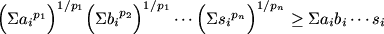MCrawford (19:31:41)
The two variable version of the inequality looks like

MCrawford (19:31:46)MCrawford (19:32:04)
Students who find this inequality difficult to work with might first go about studying the Cauchy-Schwartz and Triangle inequalities (the algrebraic version of the triangle inequality is ultimately a very specific version of Holder).

MCrawford (19:32:24)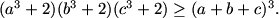MCrawford (19:32:35)
Our clues are the a^3, b^3, and c^3 terms on the left and the a + b + c on the right. It looks like powers of 3 will work for the exponents, but on the right hand side we don't want any of the variables multiplied together. This means that we can group the terms in our sequences so that each cubed variable matches up with 1^3 = 1 in the other sequences:

a^3, 1, 1,
1, b^3, 1,
1, 1, c^3.

MCrawford (19:33:03)
Letting the exponents be 3, 3, and 3 (since 1/3 + 1/3 + 1/3 = 1), we have

MCrawford (19:33:08)MCrawford (19:33:44)
Cubing and simplifying we have the desired inequality.

RK7 (19:33:10)
when you say sum(ai*bi), do you mean a1b1+a2b2 +... or a1b1+a1b2+...

MCrawford (19:34:22)
For the summations, i is the index for both sequences {a_i} and {b_i}, so each member of each sequence is multiplied with exactly one member of the other sequence.

MCrawford (19:34:45)
Let's now take a look at the hardest problem on this year's USAMO.

MCrawford (19:34:52)
6. A circle w is inscribed in a quadrilateral ABCD. Let I be the center of w. Suppose that

MCrawford (19:34:57)MCrawford (19:35:02)
How might we begin to approach this problem?

jelyman (19:35:22)
draw and label

MCrawford (19:35:31)
We could start by drawing a diagram. What features will necessarily be part of our diagram?

jelyman (19:35:53)
the circle, I, the tangency segments, the vertices

MCrawford (19:36:24)MCrawford (19:36:33)
Our diagram includes 4 points around a circle. These four points are connected in such a way that the segments AB, BC, CD, and DA are tangent to the circle meaning that the radii from I to the tangent points hit the segments at right angles. What other features can we conclude about our diagram?

jelyman (19:37:32)
the common associations of tangencies... i.e the equal segments from the tangent point

MCrawford (19:37:48)
Or we could view the arcs as equal.

MCrawford (19:38:15)MCrawford (19:38:25)
The circle is divided into 8 arcs by the lines from I to A, B, C, D, and the points of tangency. We can call the measures of these arcs a, b, c, and d. We also know that
a + b + c + d = 180 degrees.

MCrawford (19:38:30)
Now, we could work in one of many directions for a solution. What possible directions come to mind?

Nukular (19:38:53)
finding geometric representations for AI + DI, BI + CI?

jelyman (19:39:06)
comparing the lenghts to that of a right triangle

RK7 (19:39:11)
pythagoras, because we have so many right angles and sqaures in the problem statement?

MCrawford (19:39:35)
I personally looked for quite some time and never found a nice purely geometric proof for this problem.

MCrawford (19:39:43)
We have angles and right triangles. Trigonometry seems like a reasonable approach. How can we turn this into a trig problem?

Nukular (19:41:16)
we could put everything in terms of the inradius, but that doesnt look too nice

jelyman (19:41:20)
comp[aring everything in terms of the radius and the angle formed at the vertice....

schulmannerism (19:41:20)
R(2sinA + 2sinB + 2sinC + 2sinC) = sum of the sides

MCrawford (19:41:42)
We can scale the diagram so that the radius becomes 1. The equation we are given does not change after the scale.

MCrawford (19:41:52)MCrawford (19:42:04)
If we label the segments between A, B, C, D, and the points of tangency then we can rewrite the original equation as

MCrawford (19:42:14)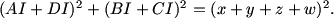jelyman (19:42:13)
where did shul's equation come from?

MCrawford (19:42:51)
He's taking the angles from the vertices. I am not.

MCrawford (19:43:06)
How else can we write this equation?

Nukular (19:43:51)
AI = x/cos (A/2)

RK7 (19:43:50)
sin(AID)^2 + ...

RK7 (19:44:00)
because AI = sin(AID)

schulmannerism (19:44:57)
we could replace AI with sqrt(1+x^2) and so forth

RK7 (19:45:05)
(sin(AID) + sin(ADI) )^2 + (sin(AIC)+sin(BCI))^2

jelyman (19:45:06)
eww?

MCrawford (19:45:41)
I personally don't want to deal with messy square roots and we have set ourselves up nicely to use the measures of the central angles instead of A, B, C, and D.

MCrawford (19:45:48)
The angles around the arcs are the same as the central angles in the circle. We can use these angles and the fact that the radius is 1 to create some nice clean trig relationships:

AI = sec(a),
BI = sec(b),
CI = sec(c),
DI = sec(d),
x = tan(a),
y = tan(b),
z = tan(c),
w = tan(d).

MCrawford (19:45:56)
We can now rewrite our equation as

MCrawford (19:46:02)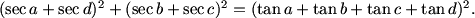MCrawford (19:46:36)
Expanding this equation we have

MCrawford (19:46:42)MCrawford (19:46:53)
How can we work with this equation?

RK7 (19:47:06)
sec^2 a-1=tan^2 a

jelyman (19:47:06)
sec^2 = 1 + tan^2

MCrawford (19:47:20)
We recall the identity sec^2(x) - tan^2(x) = 1. This gives us a natural way to collapse many terms in this equation. If we move everything over to the left hand side, collapse terms using the identity and divide by 2 we get

MCrawford (19:47:26)MCrawford (19:47:35)
Is this an equation we can work with?

schulmannerism (19:47:54)
what does the sigma subscript sym notation mean?

MCrawford (19:48:19)
It means we're summing each pair of tangents taken 2 at a time (6 times total in this case).

RK7 (19:48:08)
maybe if we look at a trigonometric statement of what we are trying to prove...?

MCrawford (19:48:57)
Good idea. What are we ultimately interested in proving?

MCrawford (19:50:01)
What do we want to prove in terms of a, b, c, and d?

pillango (19:50:07)
a=d and b=c

MCrawford (19:50:31)
Does this give us any ideas as to how we might break the left hand side up?

MCrawford (19:52:02)
do we think we want to group secasecd with tanbtand? What would be a more natural way to group the terms for good examination?

Nukular (19:52:02)
working backwards, seca sec d would be sec a ^2 and tan a tan d would be tan a ^2... we could pair them up.

MCrawford (19:52:29)
When dealing with an equation with many variables it is often a good idea to work with the equation in such a way that limits the variability. In this case we have a grouped with d and b grouped with c. Perhaps we can find a way to manipulate the variables by grouping them in the way that seems most natural:

MCrawford (19:52:35)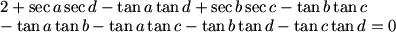MCrawford (19:52:49)
We can now group terms by factors:

MCrawford (19:52:55)MCrawford (19:53:13)
How can we work with this equation?

jelyman (19:48:08)
get rid of sec and tan for cos and sin now.

MCrawford (19:54:02)
If we break each part down into sines and cosines and apply sine and group terms into sine and cosine sum formulas we notice that

MCrawford (19:54:11)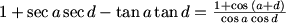MCrawford (19:54:20)MCrawford (19:54:29)MCrawford (19:54:37)
and now the left hand side of the equation looks like

MCrawford (19:54:41)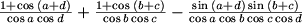MCrawford (19:55:10)
What can we now do to our equation?

RK7 (19:55:09)
multiply through by cos a * cos b * cos c * cos d because none can be zero

MCrawford (19:55:21)
We can multiply our equation through by each of the positive numbers cos(a), cos(b), cos(c), and cos(d) to get

MCrawford (19:55:27)MCrawford (19:55:36)
How many variables does the equation really have?

Xbizkitl (19:55:55)
3?

MCrawford (19:56:10)
Since a + b + c + d = 180, the equation really only has 3 variables. Does that give us any ideas as to how we might examine the equation?

pillango (19:56:57)
a+d=180-(b+c)

RK7 (19:57:09)
maybe a convexity argument...? then we'd just have to check the endpoints

MCrawford (19:57:35)
If you come up with one, please type it out.

MCrawford (19:58:27)
Pillango's idea should help us come up with a strategy. What strategies could we employ when evaluating a 3 variable equation (a single equation...with...3 variables)?

jelyman (19:56:31)
sin(b+c) = sin(a+d)

Xbizkitl (19:57:32)
We can then change that using identity like sin x = sin (180-x)?

Xbizkitl (19:58:44)
Or cos x = -cos (180 - x) use that identity to get them into a+d or b+c?

MCrawford (19:59:12)
How are those things going to help us if we still have 3 quantities that can vary?

Nukular (20:00:11)
hold b and c constant

MCrawford (20:00:23)
When dealing with numerous variables in a single equation, it is often nice to hold one or more of the variables constant.

MCrawford (20:00:41)
The problem tells us that there will be equality iff a = d and b = c. We might try holding b and c constant and seeing if there is a maximum or minimum when a = d.

MCrawford (20:00:57)
When b and c are held constant, the terms that vary are a and d, but they relate as they vary because they have a constant sum. Additionally, since we subtracted the right hand side from the left hand side of our original equation and we are looking for the left hand side to be smaller, we know that we are looking for the left hand of our most recent equation to be maximal at 0. This means that we are looking to prove that our most recent expression is nonpositive and show that the conditions that make it maximal make its value 0.

MCrawford (20:01:27)
We would like to express as much of this as possible in terms of the fixed angles b and c. We can note that sin(a + d) = sin(b + c) and cos(a + d) = -cos(b + c) which gives us

MCrawford (20:01:35)MCrawford (20:01:57)
Can we maximize the left hand side?

Nukular (20:02:43)
we only have to look at the cosacosd product.

MCrawford (20:03:05)
Since b and c are fixed, the only part of the left hand side that varies is

MCrawford (20:03:11)MCrawford (20:03:28)
Can we maximize this expression as a and d vary?

RK7 (20:04:17)
i think its maximized when a = d?

MCrawford (20:04:29)
How can we prove that?

Nukular (20:04:26)
they would be equal, since they have a constant sum.

MCrawford (20:04:38)
Why?

MCrawford (20:05:19)
How can we rewrite cos(a)cos(d)?

Nukular (20:05:22)
well we just maximize cosa * cos d. (1 + cos (b+c)) is a constant

Nukular (20:05:31)
(cos(a+d) + cos(a-d))/2

polymorphic (20:05:37)
product to sum formula

MCrawford (20:05:51)
1 + cos(b + c) is constant and nonnegative. We can maximize the left hand side of the equation by maximizing cos(a)cos(d). We can maximize this product because the sum a + d is constant. We first convert the product of cosines to a sum:

MCrawford (20:06:00)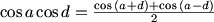Nukular (20:05:55)
cos(a+d) is constant, and cos(a-d) is maximized a=d at 1

MCrawford (20:06:11)
Since a + d is constant, so is cos(a + d). The only part that varies is cos(a - d) which is greatest when a = d and cos(a - d) = 1.

MCrawford (20:06:17)
What have we shown?

RK7 (20:06:52)
our equation is maximized when a=d, b=c

MCrawford (20:07:12)
We have shown that the left hand side of the original equation is closest to the right hand side only when a = d. Since the equation works the same way for b and c as it does for a and d, we know that the left hand side is closest to the right hand side only when b = c.

MCrawford (20:07:27)
If we let a = d and b = c = 90 - a, we can plug into any one of our equations and see that equality holds. And we are done.

MCrawford (20:08:24)
Note: There are other solutions to this problem. There is a solution that involves using Cauchy on the left hand side of the equation after expansion. Perhaps given that clue you can find that solution yourself. I'm sure the USAMO writers have prepared one or two more.

MCrawford (20:08:53)
I like this solution because it requires nothing more than a solid knowledge of trigonometry and the guts to keep working with a lot of terms.

schulmannerism (20:10:39)
wow you make these problems look so doable!

MCrawford (20:10:49)
Good question.
Honestly, I worked through 4 solutions to this last problem -- found glitches in 2 of them, and finally perfected one that I thought would be easy to motivate.

MCrawford (20:11:34)
Well, maybe not easy, but certainly doable.
I think that something I learned with experience was...
Don't be frightened of the equations.
If you can continue to manipulate, it just might get there.
Consequently I'm better with algebra than geometry.

Xbizkitl (20:12:20)
A question? How does one know when you are going too complicated, rather from beauty to messiness?

MCrawford (20:12:43)
I can't give you a perfect answer.

MCrawford (20:13:07)
My first thought was to use trig on this problem because I could scale the radius to 1 and deal 4 angles than summed to 180.
After I saw that all the lengths in the equation worked out to nice trig functions, I felt like it was a good path.
In algebra, a little bit of complication is not usually something to worry about as long as you can continue to make progress.

Xbizkitl (20:13:20)
I was suprised when you converted tans, secs, to cos and sins, because I thought the expression would become far too messy to puzzle out.

MCrawford (20:14:10)
I thought so at first too.

MCrawford (20:14:46)
But I couldn't see another way to take the equation, so I went ahead and looked at it in terms of sines and cosines. It's a natural 'second path'.

Nukular (20:13:59)
I think its just that there is nowhere else to go...

MCrawford (20:14:53)
Right.

MCrawford (20:15:24)
Often there is one way to go. The job is to find it. Typically you know when you found it and you usually know when you didn't.

MCrawford (20:15:52)
Knowing when you didn't is a skill that takes time to hone. It takes walking down a lot of blind alleys.
Though the good problem solving books often help point out the blind alleys.
We try to help with this as well.Sign In